# Underscore.js | _.sample()

The Underscore.js is a JavaScript library that provides a lot of useful functions that helps in the programming in a big way like the map, filter, invoke etc even without using any built-in objects.

The _.sample() function is used to find out what kind of elements are present in the array. It gives a random element of the array as output. We can even pass a second parameter so as to return that number of random elements from the array.

Syntax:

`_.sample(list, [n])`

Parameters:It takes two arguments:

• The List
• The number n

Return values:
It returns an of the element from the passed array.

• Passing a list of numbers to the _.sample() function:
The ._sample() function uses a random function and then displays that element from the list as the result. If the second parameter is not mentioned then t’s default value will be taken which is 1. Hence, any one of the element will be displayed.

 `` `<``html``> ` `  `  `<``head``> ` `    ``<``script` `src` `=  ` `"https://cdnjs.cloudflare.com/ajax/libs/underscore.js/1.9.1/underscore-min.js"` `> ` `    `` ` ` ` `  `  `<``body``> ` `    ``<``script` `type``=``"text/javascript"``> ` `         ``console.log(_.sample([1, 2, 3, 4, 5, 6])); ` `    `` ` ` ` `  `  ` `

Output: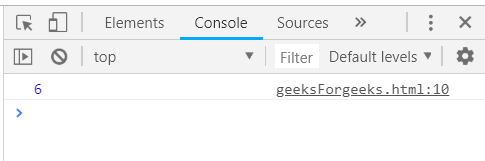• Passing the second parameter to the _.sample() function:
Id we pass the second parameter then the _.sample() function will return as many elements from the passed list as mentioned. The result will be an array containing the number of elements which is present in the second parameter.

 `` `<``html``> ` `  `  `<``head``> ` `    ``<``script` `src` `=  ` `"https://cdnjs.cloudflare.com/ajax/libs/underscore.js/1.9.1/underscore-min.js"``> ` `    `` ` ` ` `  `  `<``body``> ` `    ``<``script` `type``=``"text/javascript"``> ` `         ``console.log(_.sample([1, 2, 3, 4, 5, 6], 3)); ` `    `` ` ` ` `  `  ` `

Output: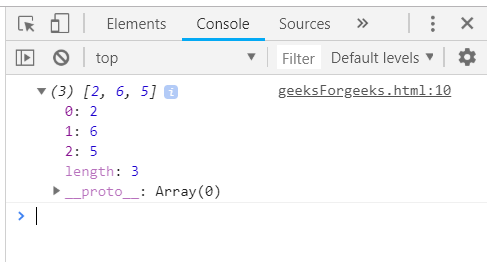• Passing a structure to the _.sample() function:
We can even pass a structure to the _.sample() function and it will work in the same manner. It will display any of the element of the structure randomly as the output. Since, no second parameter is mentioned therefore, it will have only one element of the passed list in the result along with it’s all properties.

 `` `<``html``> ` `  `  `<``head``> ` `    ``<``script` `src` `=  ` `"https://cdnjs.cloudflare.com/ajax/libs/underscore.js/1.9.1/underscore-min.js"``> ` `    `` ` ` ` `  `  `<``body``> ` `    ``<``script` `type``=``"text/javascript"``> ` `         ``var people = [ ` `        ``{"name": "sakshi", "hasLong": "false"}, ` `        ``{"name": "aishwarya", "hasLong": "true"}, ` `        ``{"name": "akansha", "hasLong": "true"}, ` `        ``{"name": "preeti", "hasLong": "true"} ` `    ``] ` `     ``console.log(_.sample(people)); ` `    `` ` ` ` `  `  ` `

Output: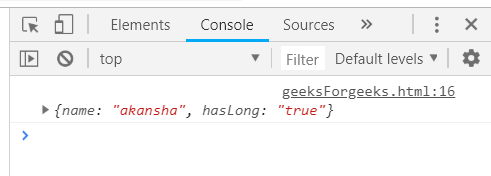• Passing a structure with only one property to the _.sample() function together:
If we pass a structure with only one property then it will work in the same way and display any one of the element randomly from the structure passed. Here also, since the second parameter is not mentioned therefore, the resultant array will contain only one element.

 `` `<``html``> ` `  `  `<``head``> ` `    ``<``script` `src` `=  ` `"https://cdnjs.cloudflare.com/ajax/libs/underscore.js/1.9.1/underscore-min.js"``> ` `    `` ` ` ` `  `  `<``body``> ` `    ``<``script` `type``=``"text/javascript"``> ` `         ``var users = [{"num":"10"}, {"num":"9"},  ` `{"num":"8"}, {"num":"7"}, {"num":"6"}]; ` `    ``console.log(_.sample(users)); ` `    `` ` ` ` `  `  ` `

Output: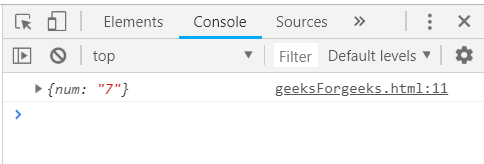NOTE:
These commands will not work in Google console or in firefox as for these additional files need to be added which they didn’t have added.

 `` `<``script` `type``=``"text/javascript"` `src` `= ` `"https://cdnjs.cloudflare.com/ajax/libs/underscore.js/1.9.1/underscore-min.js"``> ` ` `

An example is shown below: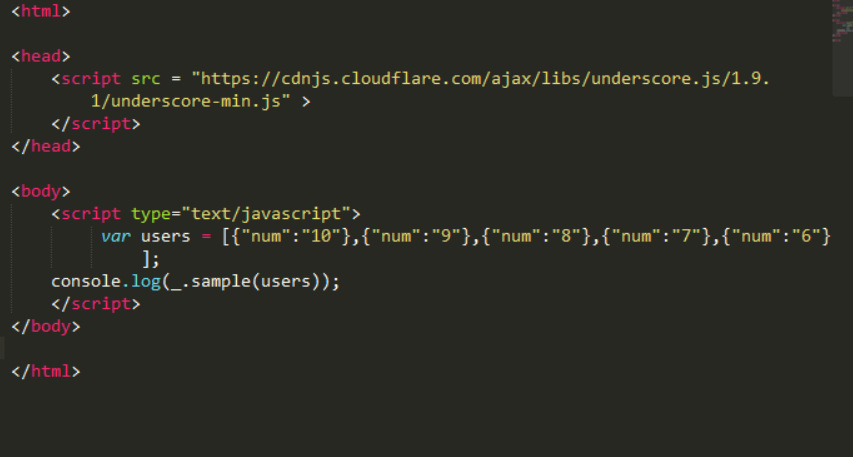My Personal Notes arrow_drop_upI like to do coding in C++C and java programming languages HTML and CSS always intersts me Sharing knowleged is the best way according to me to increase ones knwoledge

If you like GeeksforGeeks and would like to contribute, you can also write an article using contribute.geeksforgeeks.org or mail your article to contribute@geeksforgeeks.org. See your article appearing on the GeeksforGeeks main page and help other Geeks.

Please Improve this article if you find anything incorrect by clicking on the "Improve Article" button below.

Article Tags :

Be the First to upvote.

Please write to us at contribute@geeksforgeeks.org to report any issue with the above content.